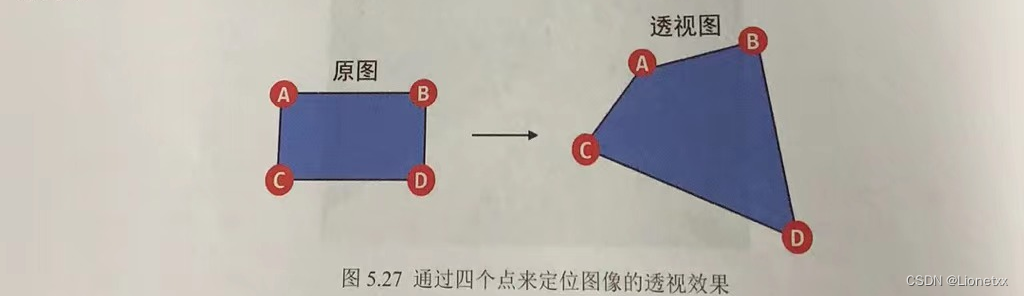# 图像的放大与缩小

• `src`：要缩放的图片
• `dsize`：缩放之后的图片大小，用元组和列表表示均可，如果按照xy轴比例进行放缩，则要写出`dsize = None`
• `dst`：可选参数，缩放之后的输出图片(一般用不上)
• `fx,fy`x轴和y轴的缩放比，即宽度和高度的缩放比
• `interpolation`：插值算法——> 例如当图片被放大时，我们怎么去填充空白区域，就用到了此算法，其中：

• `INTER_NEAREST`：邻近插值，速度快，效果差
• `INTER_LINEAR`：双线性插值，使用原图中的四个点进行插值**(默认方法）**
• `INTER_CUBIC`：三次插值，使用原图中的16个点进行插值
• `INTER_AREA`：区域插值，效果最好，计算时间最长

## 放缩到指定大小：

``````import cv2
import numpy as np

# 读取图片

# 读取图片大小
print("cat.shape：",cat.shape) # (480, 640, 3)
print("dog.shape：",dog.shape) # (360, 499, 3)   # shape函数显示:（行(高度)，列(宽度)，通道数）

#把猫缩放成和狗一样大 ——> 注意opencv中的显示：先是宽度，再是高度，而我们正常显示shape时是先行(高度)后列(宽度)
new_cat = cv2.resize(cat,(499,360)) # 要反过来！

print("new_cat.shape：",new_cat.shape) # (360, 499, 3)

cv2.imshow('new_img',np.hstack((new_cat,dog))) # 如果能成功拼到一起，则修改成功！

cv2.waitKey()
cv2.destroyAllWindows()
``````

``````cat.shape： (480, 640, 3)
dog.shape： (360, 499, 3)
new_cat.shape： (360, 499, 3)
``````## 根据xy轴的比例进行放缩：

``````import cv2
import numpy as np

# 读取图片

# 读取图片大小
print("cat.shape：",cat.shape) # (480, 640, 3)
# shape函数显示:（行(高度)，列(宽度)，通道数）

# 还可以按照x,y轴的比例进行缩放 ——> 此时要把参数dsize写成None
new_cat = cv2.resize(cat,dsize = None,fx = 0.5,fy = 0.5)

print("new_cat.shape：",new_cat.shape) # (360, 499, 3)

cv2.imshow('cat',cat)
cv2.imshow('new_cat',new_cat)

cv2.waitKey()
cv2.destroyAllWindows()
``````

``````cat.shape： (480, 640, 3)
new_cat.shape： (240, 320, 3)
``````# 仿射变换

• `M`变换矩阵
• `dsize`输出图片大小——> 最好和原图大小一致
• `flag`：与`resize()`中的插值算法一致 （用默认值即可）
• `mode`：边界外推法标志，即当图像超出边界时该怎么办 （此处用默认值即可）
• `value`：填充边界值 （用默认值即可）

## 仿射变换之图像的平移`tx = 沿x轴方向向右平移的距离(向左为负) ；ty = 沿y轴方向向下平移的距离(向上为负) ——原点在图片左上角`

``````# 将图片向右平移200个像素点

import cv2
import numpy as np

# 打印图像cat的高度、宽度，便于设置参数dsize
h,w,ch = cat.shape # 注意！shape()输出是先高度(行)，后宽度(列)

# 变换矩阵 ——> 要求矩阵类型最小是float32位
M = np.float32([ [1,0,200],[0,1,0] ])

# 平移操作
new_cat = cv2.warpAffine(cat,M,dsize = (w,h)) # dsize为输出图片的大小，注意！opencv是先宽度(列)，后高度(行)，要反过来

cv2.imshow('new_cat',new_cat)
cv2.imshow('cat',cat)

cv2.waitKey()
cv2.destroyAllWindows()
``````## 仿射变换之获取变换矩阵`M`

### 方法一：利用旋转角度获取变换矩阵

``````# 在进行图像的旋转操作时，手动计算变换矩阵不太方便，而opencv提供了获取旋转变换矩阵的API
import cv2
import numpy as np

# 打印图像cat的高度、宽度，便于设置参数dsize
h,w,ch = dog.shape # 注意！shape()输出是先高度(行)，后宽度(列)

# 获取变换矩阵 变换矩阵一定是2D的(二维)
m = cv2.getRotationMatrix2D((100,100),15,1) #以 (100,100)为原点（左上角为(0，0)）；逆时针旋转15°；scale = 1表示不缩放

new_dog = cv2.warpAffine(dog,m,(w,h))

cv2.imshow('new_dog',new_dog)
cv2.imshow('dog',dog)

cv2.waitKey()
cv2.destroyAllWindows()
``````### 方法二：利用变换前后对应点的关系获取变换矩阵

• `src`：原目标的三个点`src = np.float32([ [x1 , y1] , [x2 , y2] , [x3 , y3] ])`
• `dst`：对应变换后的三个点`dst = np.float32([ [x^1 , y^1] , [x^2 , y^2] , [x^3 , y^3] ])```````# 通过三个点来确定变换矩阵

import cv2
import numpy as np

# 打印图像cat的高度、宽度，便于设置参数dsize
h,w,ch = cat.shape # 注意！shape()输出是先高度(行)，后宽度(列)

# 获取变换矩阵：需要原始图片的三个点坐标 "src"，和变换之后对应的三个点的坐标 "dst"
src = np.float32([ [200,100] , [300,100] , [200,300]])
dst = np.float32([ [100,150] , [360,200] , [280,120]])
m = cv2.getAffineTransform(src,dst)

new_cat = cv2.warpAffine(cat,m,(w,h))

cv2.imshow('new_cat',new_cat)
cv2.imshow('cat',cat)

cv2.waitKey()
cv2.destroyAllWindows()
``````(变换得雀食抽象，我也没去仔细算，会用就行?)

# 透视变换`OpenCV`中需要通过定位图像的四个点来进行计算透视效果，四个点的位置如图，`OpenCV`根据四个点的位置变化来计算出其他像素的位置变化透视效果不能保证图像的“平直性”和“平行性”• `src`：变换前图片的四个点**(随意选取)**，但一般我们会选择原图片的四个顶点
• `dst`：变换后图片的四个点，和`src`一样，我们一般选择新图片的四个顶点

• `src`：原图
• `M`变换矩阵，对于透视矩阵来说，M是一个3*3的矩阵
• `dsize`输出图片大小——> 最好和原图大小一致
• `flag`：与`resize()`中的插值算法一致 （用默认值即可）
• `mode`：边界外推法标志，即当图像超出边界时该怎么办 （此处用默认值即可）
• `value`：填充边界值 （用默认值即可）
``````# 仿射变换——> 对坐标系的变换，通过原先图片的四个点和变换后图片的四个点构造方程组

# 在此例，我们相当于用仿射变换把书中这一页抠出来并且摆正

import cv2
import numpy as np

# 我们图片大小为宽1276×高1702

# 打印图像cat的高度、宽度，便于设置图像中的四个点和设置参数dsize
h,w,ch = book.shape # 注意！shape()输出是先高度(行)，后宽度(列)

# 获取变换矩阵 ——> 顺序：左上，左下，右上，右下
# src = np.float32([[200,200],[1700,100],[200,1000],[1700,1200]])
# dst = np.float32([[0,0],[1700,0],[0,1100],[1700,1100]])   # 格式为[h,w]，在图像中横轴是w，纵轴是h

# 获取变换矩阵 ——> 顺序：左上，,右上，左下，右下
src = np.float32([[200,200],[50,1000],[800,0],[700,1100]])
dst = np.float32([[0,0],[0,1100],[900,0],[900,1100]])
m = cv2.getPerspectiveTransform(src,dst)

# 透视变换
new_book = cv2.warpPerspective(book,m,(900,1100))

# 由于我们的图太大了，需要再设置窗口大小
cv2.namedWindow('new_book',cv2.WINDOW_NORMAL)
cv2.resizeWindow('new_book',640,480)
cv2.imshow('new_book',new_book)

cv2.namedWindow('book',cv2.WINDOW_NORMAL)
cv2.resizeWindow('book',640,480)
cv2.imshow('book',book)

cv2.waitKey()
cv2.destroyAllWindows()
``````THE END)">